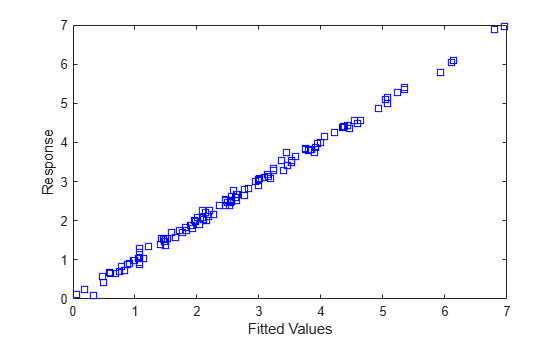# response

Class: LinearMixedModel

Response vector of the linear mixed-effects model

## Syntax

``y = response(lme)``

## Description

example

````y = response(lme)` returns the response vector `y` used to fit the linear mixed-effects model `lme`.```

## Input Arguments

expand all

Linear mixed-effects model, specified as a `LinearMixedModel` object constructed using `fitlme` or `fitlmematrix`.

## Output Arguments

expand all

Response values, specified as an n-by-1 vector, where n is the number of observations.

Data Types: `single` | `double`

## Examples

expand all

`load('weight.mat');`

`weight` contains data from a longitudinal study, where 20 subjects are randomly assigned to 4 exercise programs, and their weight loss is recorded over two-week time periods. This is simulated data.

Store the data in a table. Define `Subject` and `Program` as categorical variables.

```tbl = table(InitialWeight,Program,Subject,Week,y); tbl.Subject = nominal(tbl.Subject); tbl.Program = nominal(tbl.Program);```

Fit a linear mixed-effects model where the initial weight, type of program, week, and the interaction between the week and type of program are the fixed effects. The intercept and week vary by subject.

`lme = fitlme(tbl,'y ~ InitialWeight + Program*Week + (Week|Subject)');`

Compute the fitted values and the response.

```F = fitted(lme); y = response(lme);```

Plot the response versus the fitted values.

```plot(F,y,'bs') xlabel('Fitted Values') ylabel('Response')```